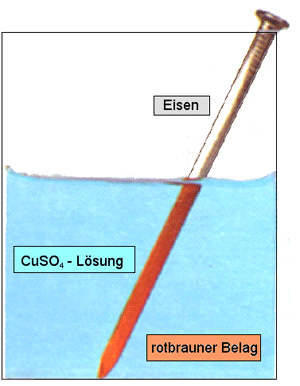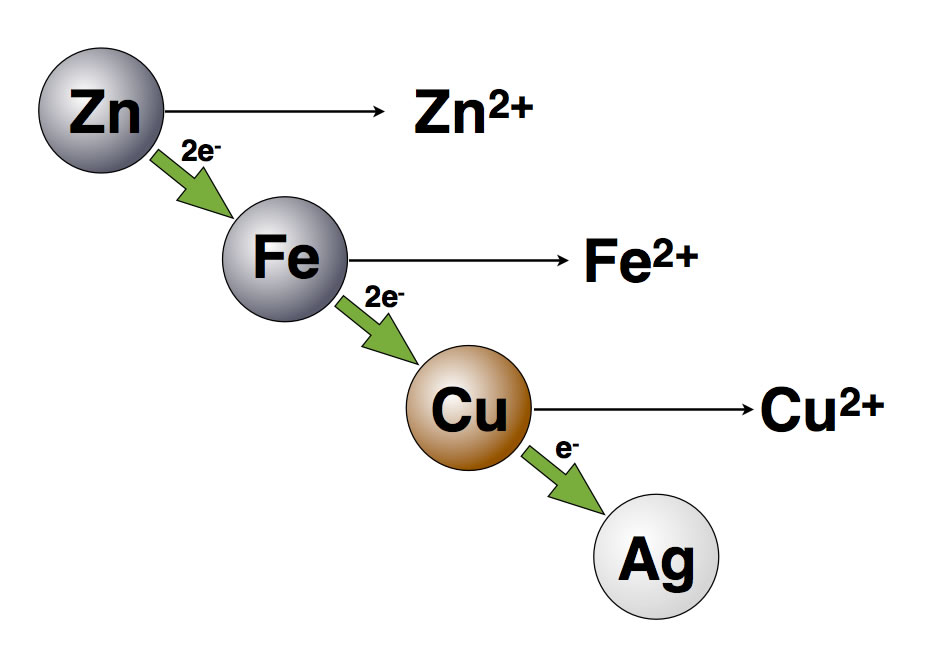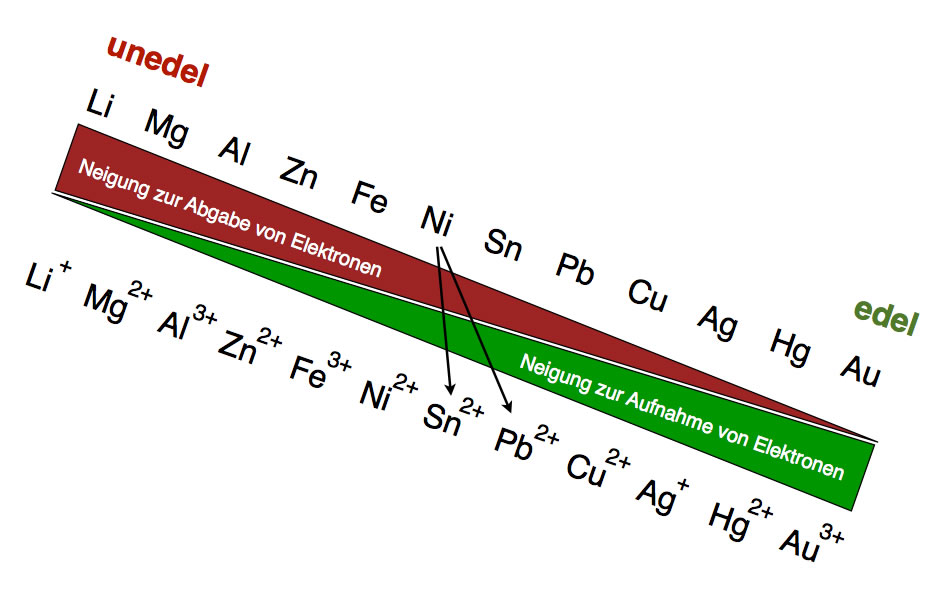# Silver nitrate oxidizes copper

## Redox series of metals S1, EF, Q1

### The iron nail attempt

First of all, let's make a simple but impressive attempt:

attempt

Iron nail in copper sulfate
###### Execution:

We put a copper sulfate solution in a beaker (100 ml) and put a large iron nail in it. We watch the iron nail for a long time.

###### Observations:After a while, the iron nail was covered with a red-brown coating.

This red-brown coating is not rust, but pure copper. The following redox reaction has taken place:

$Cu ^ {2 +} _ {(aq)} + Fe _ {(s)} \ to Fe ^ {2 +} _ {(aq)} + Cu _ {(s)}$

If the browser does not display the formulas on this page correctly, the MathJax extension was not loaded correctly. Either the browser is out of date or there is currently no internet connection.

In the redox reactions discussed so far, a metal such as sodium or magnesium has always reacted with a non-metal such as chlorine or oxygen. The metal was the electron donor and gave up one, two or three electrons to the non-metal, which played the role of the electron acceptor.

The reaction between iron and copper sulfate does not fit into this scheme. Here one metal reacts with the ions of another metal.

The iron atoms give electrons to the copper ions, so that iron ions and copper atoms are formed. Obviously, copper has a greater "need" to accept electrons than iron, while iron has a greater "propensity" to donate electrons than copper.

Let us set up the classic partial equations for the above redox reaction, i.e. first the oxidation, then the reduction and finally the overall reaction, which is then referred to as the redox reaction:

$Fe _ {(s)} \ to Fe ^ {2 +} _ {(aq)} + 2 e ^ {-} \ \ \ \ (Oxidation)$

$Cu ^ {2 +} _ {(aq)} + 2 e ^ {-} \ to Cu _ {(s)} \ \ \ \ (reduction)$

---------------------------------------------------------------

$Cu ^ {2 +} _ {(aq)} + Fe _ {(s)} \ to Fe ^ {2 +} _ {(aq)} + Cu _ {(s)} \ \ \ \ (redox reaction)$

A numerical adjustment of the two equations is not necessary, since exactly as many electrons are released during the oxidation as are required during the reduction.

### Further attempts of this kind

If we put a sheet of copper in an iron sulfate solution, nothing happens. An electron transfer from the copper to the iron ions does not take place, obviously this electron transfer is a "one-way street". The electrons only flow from the iron to the copper ions, but not from the copper to the iron ions.

attempt
###### Execution:
1. We fill a 50 ml beaker with silver nitrate solution and then place a clean copper sheet in the solution.
2. We observe over a longer period of time.
###### Observations:

Here again there is a clear reaction. The copper sheet turns black!

The redox reaction that takes place in this experiment looks like this:

$2 Ag ^ {+} _ {(aq)} + Cu _ {(s)} \ to Cu ^ {2 +} _ {(aq)} + 2 Ag ^ {+} _ {(s)}$

The copper atoms give electrons to the silver ions. The reaction does not work in the opposite direction. Silver atoms are "reluctant" to give up electrons, while silver ions are "happy" to accept electrons. That's the way it is with precious metals. Base metals such as sodium or magnesium are "very happy" to give up electrons, while the ions of base metals are "reluctant" to take up electrons again.

You can now carry out further experiments of this kind (and do that in school lessons too). If, for example, an iron nail is placed in a silver nitrate solution, a coating of pure silver is formed here as well. And if you put magnesium in an iron sulphate solution, an iron layer forms on the magnesium, because iron is more noble than magnesium and the Mg atoms therefore "like" to give electrons to the iron ions.

If you have carried out many such experiments systematically, you come to the so-called redox series of metals, which is represented graphically as follows:In this drawing, however, only four metals have been taken into account. Zinc is the least noble, iron is less noble than copper, and copper is less noble than silver. Magnesium, for example, is even less noble than zinc, while gold is even more noble than silver. In this way, all metals that exist can be accommodated in this redox series. Those who are more interested can go to the page that was written for high school courses.Finally, an expanded version of the redox series with twelve metals. With the help of such a graphic one can see chemical reactions predict. For example, if you put a zinc sheet (Zn) in a lead sulfate solution, you get a reaction; a lead layer forms around the zinc sheet. Lead (Pb) is more noble than zinc, so it "likes" to accept electrons. If, on the other hand, a lead sheet is placed in a zinc sulphate solution, there is no reaction.

### Summary: Reactions between metal atoms and metal ions

A common chemical reaction is the transfer of electrons from one metal to the cations of another metal. This electron transfer is an example of donor-acceptor reactions.

The tendency to give up electrons differs from metal to metal. The base metals give off electrons relatively easily, while the noble metals prefer to keep their electrons. If you order the metals according to their decreasing tendency to donate electrons, you get the redox series of the metals - here is a small excerpt:Less noble metals can only give their electrons "downhill" to the cations of more noble metals; zinc, for example, can give electrons to iron ions, copper ions or silver ions. The reverse route "uphill" is not possible, for example copper cannot give up electrons to iron ions.